Students can download Maths Chapter 5 Information Processing Ex 5.2 Questions and Answers, Notes, Samacheer Kalvi 6th Maths Guide Pdf helps you to revise the complete Tamilnadu State Board New Syllabus, helps students complete homework assignments and to score high marks in board exams.

## Tamilnadu Samacheer Kalvi 6th Maths Solutions Term 3 Chapter 5 Information Processing Ex 5.2

Miscellaneous Practice Questions

Question 1.
Find HCF of 188 and 230 by Euclid’s game.
Solution:
By Euclid’s game HCF (a, b) = HCF (a, a – b) if a > b.
Here HCF (188, 230) = HCF (230, – 188) because 230 > 188
= HCF (188, 42) = HCF (146, 42)
= HCF (104, 42) = HCF (62, 42)
= HCF (42, 20) = HCF (22, 20)
= HCF (20,2) = HCF (18, 2) = 2
∴ HCF (230, 188) = 2Question 2.
Write the numbers from 1 to 50. From that find the following.
i) The numbers which are neither divisible by 2 nor 7.
ii) The prime numbers between 25 and 40
iii) All square numbers upto 50.
Solution:
1, 2, 3, 4, 5, 6, 7, 8, 9, 10, 11, 12, 13, 14, 15, 16, 17, 18, 19, 20, 21, 22, 23, 24, 25, 26, 27, 28, 29, 30, 31, 32, 33, 34, 35, 36, 37, 38, 39, 40, 41, 42, 43, 44, 45, 46, 47, 48, 49, 50.
i) The numbers neither divisible by 2 nor 7 are 9, 11, 13, 15, 17, 19, 23, 25, 27, 29, 31, 33, 37, 39, 41, 43, 45, 47.
ii) The prime numbers between 25 and 40 are 29, 31, 37.
iii) Square numbers upto 50 are 1, 4, 9, 16, 25, 36, 49

Question 3.
Complete the following pattern.
(i) 1 + 2 + 3 + 4 = 10
2 + 3 + 4 + 5 = 14
___ + 4 + 5 + 6 = ___
4 + 5 + 6 + ___ = ___

(ii) 1 + 3 + 5 + 7 = 16
___ + 5 + 7 + 9 = 24
5 + 7 + 9 + ___ = ___
7 + 9 + ___ + 13 = ___

(iii) AB, DEF, HIJK, ___ , STUVWX
(vi) 20, 19, 17, ___ , 10, 5
Solution:
(i) 3, 18; 7, 22
(ii) 3; 11, 32; 11, 40
(iii) MNOPQ
(iv) 14Question 4.
Complete the table by using the following instructions.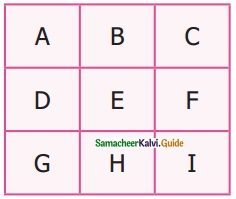A : It is the 6th term in the Fibonacci sequence.
B : The predecessor of 2.
C : LCM of 2 and 3.
D : HCF of 6 and 20.
E : The reciprocal of 1/5.
F : The opposite number of -7.
G : The first composite number.
H : Area of a square of side 3 cm.
I : The number of lines of symmetry of an equilateral triangle.
After completing the table, what do you observe? Discuss.
Solution:
A – 8, B – 1, C – 6, D – 2, E – 5, F – 7, G – 4, H – 9, I – 3Question 5.
Assign the number for English alphabets as 1 for A, 2 for B upto 26 for Z. Find the meaning of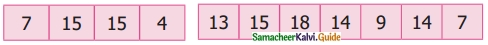Solution:
GOOD MORNING

Question 6.
Replace the letter with symbols as + for A, – for B, × for C, and ÷ for D. Find the answer for the pattern 4B3C5A30D2 by doing the given operations.
Solution:
Given the symbols + for A; – for B; × for C; + for D .
∴ 4B3C5A30D2 becomes
4 – 3 × 5 + 30 ÷ 2 Using BIDMAS rule
4 – 3 × 5 + 30 ÷ 2 = 4 – 3 × 5 + 15[× done first]
= 4 – 15 + 15 [+ done second]
= 4 – 0 [+ done third]
= 4 [- done last]

Question 7.
Observe the pattern and find the word by hiding the Numbers 1 H 2 0 3 W, 4 A 5 R 6 E, 7 Y 8 0 9 U.
Solution:
HOW ARE YOUQuestion 8.
Arrange the following from the eldest to the youngest. What do you get?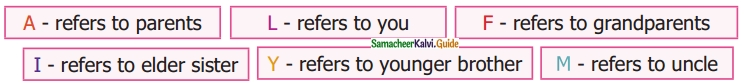Solution:
Arranging from eldest to the youngest we get
F – refers to grandparents
A – refers to parents
M – refers to an uncle
I – refers to elder sister
L – refers to me
Y – refers to the younger brother
So we get FAMILY

Challenge Problems

Question 9.
Prepare a daily time schedule for evening study at home.
Solution:
5.00 pm to 6.00 pm – Mathematics
6.0 pm to 7.00 pm – Science
7.0 pm to 8.00 pm – Social Science
8. pm to 9.00 pm – Dinner & Recreation
9. pm to 10.00 pm – Tamil and EnglishQuestion 10.
Observe the geometrical pattern and answer the following questions.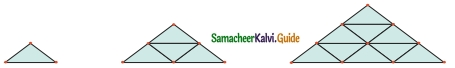(i) Write down the number of sticks used in each iterative pattern,
(ii) Draw the next figure in the pattern also find the total number of sticks used in it.
Solution:
(i) 3, 9, 18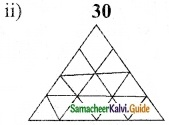Question 11.
Find the HCF of 28, 35, 42 by Euclid’s game.
Solution:
HCF of 28, 35, 42
HCF of (28, 35 – 28, 42 – 28)
28 = 2 × 2 × 7
7 = 1 × 7
14 = 2 × 7
HCF of (28, 7, 14) = 7

Question 12.
Follow the given instructions to fill your name in the OMR sheet.
1. The name should be written in capital letters from left to right.
2. One alphabet is to be entered in each box.
3. If any empty boxes are there at the end they should be left blank.
4. Ballpoint pen is to be used for shading the bubbles for the corresponding alphabets.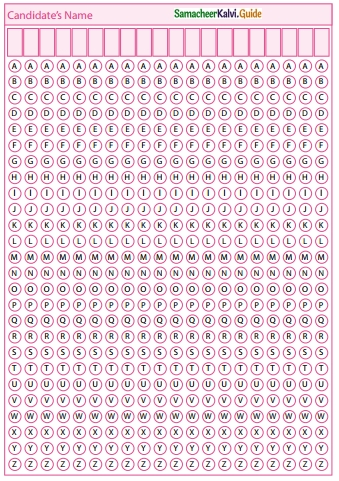Solution: